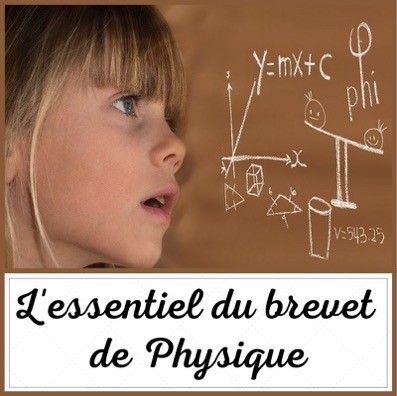# PHYSICAL PATENT: THE ESSENTIAL

III. ELECTRICITY :

III. 1. The voltage-current relationship: Ohm's law

Ohm's law is a empirical physical law which relates the intensity of the electric current passing through an electric dipole to the voltage at its terminals. This law makes it possible to determine the value of a resistance.

where U (the voltage) is in Volts (V); R (the resistance) is in Ohms and I the intensity in Amps (A).

La resistance R is the ability of an electrical conductor to pass electrical current.

L'intensity I of the electric current is the amount of electricity that passes through an electrical device in one second.

By extension :

Examples : an ohmic conductor has a resistance of 50 Ohms and is traversed by a current of 2 A of intensity. The voltage at its terminals is 50 times 2 or 100 V.

III.2. Electric power:

La electric power is the product of the electric voltage across which the device is connected and the intensity of the electric current flowing through it.

where P the electric power is in Watt (W); the voltage U is in Volts (V) and the intensity I is in Amperes (A).

La tension U at the terminals of an electrical device is the difference in electrical state that exists between the input and the output of the device.

By extension :

Examples : An electrical device has at its terminals a voltage of 25 V. It is traversed by a current of electrical intensity of 2 A. The value of the electrical power received by this device is 25 times 2 or 50 W

III.3. Electric energy:

THEelectric energy is energy related to electrical phenomena such as the flow of electric current.

where E (energy) is in Joule (J), voltage P is in watt (W) and the duration of use t is in s.

Examples : A 10 kW electric washing machine operates for 3 hours. The value of the energy used is 10 times 3 or 30 kWh

Quote on physics:

“A modern physicist studies quantum physics on Mondays, Wednesdays and Fridays and meditates on the theory of gravitational relativity on Tuesdays, Thursdays and Saturdays. On Sunday he prays… that someone will find the correlation between the two. ”

Norbert Wiener

Similar articles:

Maths certificate: the essentials